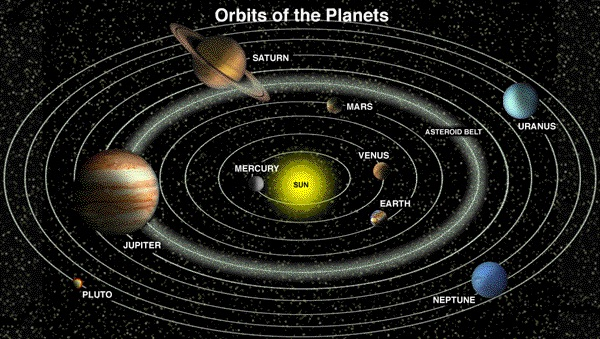# Propositions of AstronomyPlanets (condensed matter) in Space (dispersed matter)
(click to enlarge)

PROPOSITION I

The respective and relative motion of three gravitating bodies revolving together and about each other, is as four to three, or one and one-third of one primary circumference.

First Proportion: As one primary circumference of a circle is to the moon's time about the earth, so is the moon's time about the earth to the earth's time about the sun.

Second Proportion: The square of the diameter of the moon is to the square of the diameter of the earth, as the moon's time around the earth is to the earth's time round the sun, - the time here meant being circular time, as before.

Proposition II

The moon's orbit (or moon's time) round the earth in a sidereal lunation, over the value of a complete circle, is one and one-third of one primary circumference.

Proposition III

The earth's time round the sun over the value of a complete circle in space is as four to three, or one and one-third the moon's time round the earth over the value of a complete circle in space.

Proposition IV

First Proportion: As one primary circumference of a circle is to the moon's time about the earth over the value of a complete circle in space, so is the moon's time round the earth to the earth's time round the sun over the value of a complete circle in space.

Proposition V

Second Proportion: The square of the diameter of the moon is to the square of the diameter of the earth, as the moon's time round the earth over the value of a complete circle in space, is to the earth's time round the sun over the value of a complete circle in space.

Proposition VI

The mean distance of the sun's center from the center of the earth, or that at which the earth would revolve, if the area or plane of her elliptical orbit were made the area of a circle, is eleven thousand six hundred and sixty four diameters of the earth, neither more nor less; admitting, therefore, that the earth's diameter is 7,912 English miles (which no doubt is pretty nearly), then the sun's center is distant from the earth's center as above 92,285,568 English miles, and neither one mile more or less.

Axioms as proven herein and as self-evident: First: The circle is the basis or beginning of all magnitude or area. Second: Any expression of numbers in relation to material things is also an expression of magnitude. Third: A point is therefore a magnitude when considered as one. Fourth: A point in reference to space or extension on all sides of it, is therefore a molecule or globe, and in reference to a plane, it is a circle.